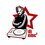# An apparent fallacy

If we want to integrate

$$\LARGE \int \frac{dx}{xlnx}$$

We may use the integration by parts technique instead of substitution to get $\LARGE I=\int { \frac { dx }{ x\ln { x } } } =\frac { 1 }{ \ln { x } } \int { \frac { dx }{ x } } -\int { \left( -\frac { 1 }{ { (\ln { x } ) }^{ 2 } } \frac { 1 }{ x } \int { \frac { dx }{ x } } \right) dx }\\ \\ \LARGE=\frac { 1 }{ \ln { x } } \ln { x } +\int { \frac { dx }{ x\ln { x } } }\\ \\ \LARGE=1+I \\ \\ \LARGE\Rightarrow I=I+1\Rightarrow 1=0$

Which is (of course)wrong.

What we should see here is the importance of the arbitrary constant of integration $\Large C$.

So if the constant of integration on the left hand side is $\Large C_1$ and on the right hand side the constant is $\Large C_2$, we may see that

$\LARGE C_1-C_2=1$

Which resolves the fallacy.

We might well see that the functions on both the right and left hand side have the same derivative i.e. $\frac{1}{x\ln(x)}$ ,proving that we are right.

We might have many interesting apparent "fallacies" as mentioned in this post .Note by Krishna Jha
6 years, 9 months ago

This discussion board is a place to discuss our Daily Challenges and the math and science related to those challenges. Explanations are more than just a solution — they should explain the steps and thinking strategies that you used to obtain the solution. Comments should further the discussion of math and science.

When posting on Brilliant:

• Use the emojis to react to an explanation, whether you're congratulating a job well done , or just really confused .
• Ask specific questions about the challenge or the steps in somebody's explanation. Well-posed questions can add a lot to the discussion, but posting "I don't understand!" doesn't help anyone.
• Try to contribute something new to the discussion, whether it is an extension, generalization or other idea related to the challenge.

MarkdownAppears as
*italics* or _italics_ italics
**bold** or __bold__ bold
- bulleted- list
• bulleted
• list
1. numbered2. list
1. numbered
2. list
Note: you must add a full line of space before and after lists for them to show up correctly
paragraph 1paragraph 2

paragraph 1

paragraph 2

[example link](https://brilliant.org)example link
> This is a quote
This is a quote
    # I indented these lines
# 4 spaces, and now they show
# up as a code block.

print "hello world"
# I indented these lines
# 4 spaces, and now they show
# up as a code block.

print "hello world"
MathAppears as
Remember to wrap math in $$ ... $$ or $ ... $ to ensure proper formatting.
2 \times 3 $2 \times 3$
2^{34} $2^{34}$
a_{i-1} $a_{i-1}$
\frac{2}{3} $\frac{2}{3}$
\sqrt{2} $\sqrt{2}$
\sum_{i=1}^3 $\sum_{i=1}^3$
\sin \theta $\sin \theta$
\boxed{123} $\boxed{123}$